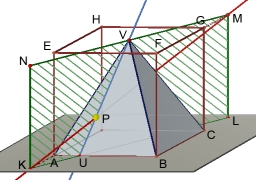# Calculate 8354

In a regular pyramid in which the edge of the base is | AB | = 4cm; height = 6cm, calculate the angle of the lines AV and CV, V = vertex.

X =  50.4788 °

### Step-by-step explanation:Did you find an error or inaccuracy? Feel free to write us. Thank you!

Tips to related online calculators
Pythagorean theorem is the base for the right triangle calculator.